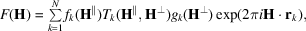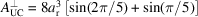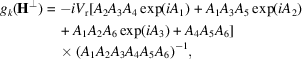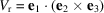International
Tables for
Crystallography
Volume B
Reciprocal space
Edited by U. Shmueli

International Tables for Crystallography (2010). Vol. B, ch. 4.6, p. 619   | 1 | 2 |

## Section 4.6.3.3.3.3. Structure factor

W. Steurera* and T. Haibacha

aLaboratory of Crystallography, Department of Materials, ETH Hönggerberg, HCI G 511, Wolfgang-Pauli-Strasse 10, CH-8093 Zurich, Switzerland
Correspondence e-mail:  walter.steurer@mat.ethz.ch

#### 4.6.3.3.3.3. Structure factor

| top | pdf |

The structure factor of the icosahedral phase corresponds to the Fourier transform of the 6D unit cell,with 6D diffraction vectors, parallel-space atomic scattering factor, temperature factorand perpendicular-space geometric form factor.is equivalent to the conventional Debye–Waller factor anddescribes random fluctuations in perpendicular space. These fluctuations cause characteristic jumps of vertices (phason flips) in the physical space. Even random phason flips map the vertices onto positions that can still be described by physical-space vectors of the type. Consequently, the setof all possible vectors forms amodule. The shape of the atomic surfaces corresponds to a selection rule for the positions actually occupied. The geometric form factoris equivalent to the Fourier transform of the atomic surface, i.e. the 3D perpendicular-space component of the 6D hyperatoms.

For the example of the canonical 3D Penrose tiling,corresponds to the Fourier transform of a triacontahedron:whereis the volume of the 6D unit cell projected uponandis the volume of the triacontahedron.andare equal in the present case and amount to the volumes of ten prolate and ten oblate rhombohedra:. Evaluating the integral by decomposing the triacontahedron into trigonal pyramids, each one directed from the centre of the triacontahedron to three of its corners given by the vectors, one obtainswithrunning over all site-symmetry operations R of the icosahedral group,,,,,andthe volume of the parallelepiped defined by the vectors(Yamamoto, 1992b).

### References

Yamamoto, A. (1992b). Ideal structure of icosahedral Al–Cu–Li quasicrystals. Phys. Rev. B, 45, 5217–5227.